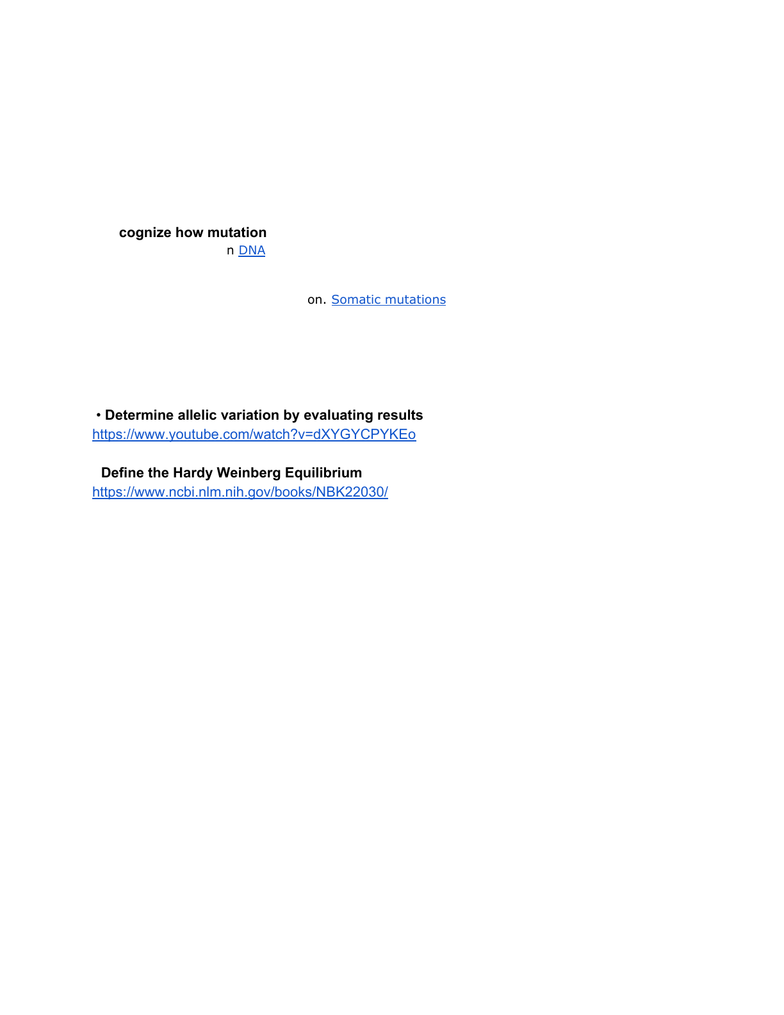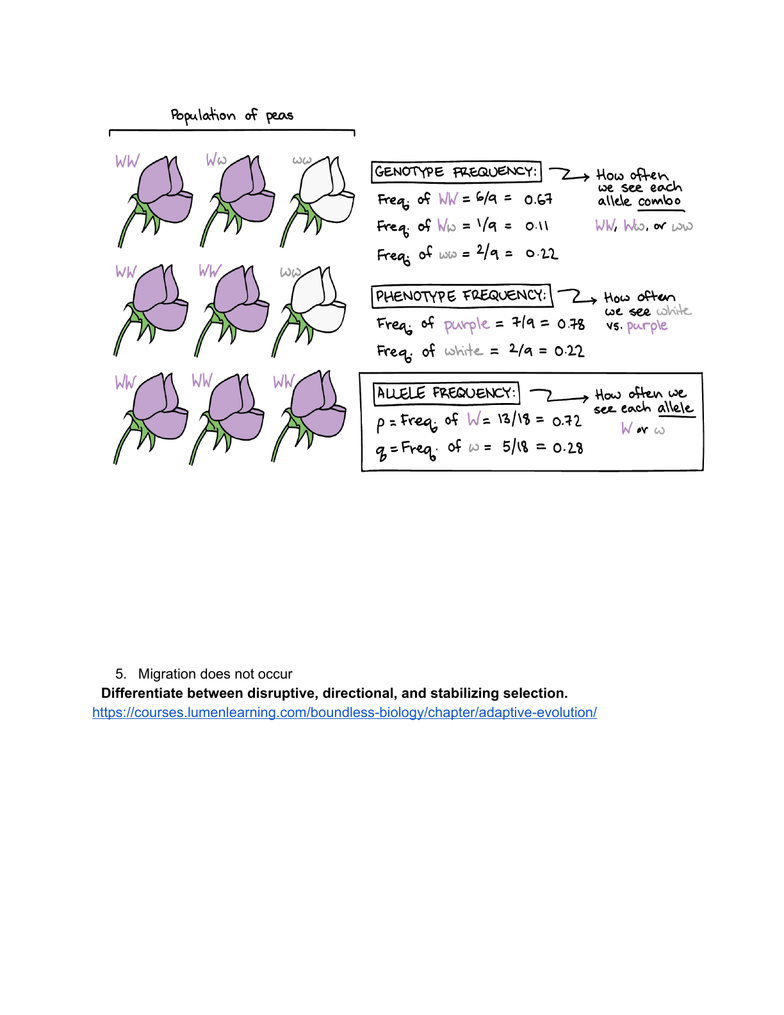Study Guides (380,000)
US (220,000)
UCLA (3,000)
LIFESCI (200)
Midterm

LIFESCI 7B Study Guide - Midterm Guide: Genotype Frequency, Allele Frequency, Genetic Variation

Department
Life Sciences
Course Code
LIFESCI 7B
Professor
princess gillbert
Study Guide
Midterm

This preview shows pages 1-3. to view the full 30 pages of the document.Ya’ll got this!!!!!!!!
Midterm 2 review
Learning objectives wks 4-7 :
Week 4:
Recognize how mutation and recombination are the two sources of genetic variation
Mutation is a change in DNA, thus causing genetic variation. These changes are random, such as
the altering of gene number or position
Since all cells in our body contain DNA, there are lots of places for mutations to occur; however,
not all mutations matter for evolution. Somatic mutations occur in non-reproductive cells and
won't be passed onto offspring.
Genetic variation can be caused by mutation (which can create entirely new alleles in a population , random
mating, random fertilization, and recombination between homologous chromosomes during meiosis (which
reshuffles alleles within an organism’s offspring . (random mating, random fertilization, and recombination all
result in different allele combinations or increased genetic variation
Determine allelic variation by evaluating results shown on a gel.
Define the Hardy Weinberg Equilibrium
https://www.ncbi.nlm.nih.gov/books/NBK22030/
The model has five basic assumptions: 1 the population is large (i.e., there is no genetic drift ; 2
there is no gene flow between populations, from migration or transfer of gametes; 3 mutations
are negligible; 4 individuals are mating randomly; and 5 natural selection is not operating on
the population. Given these assumptions, a population's genotype and allele frequencies will
remain unchanged over successive generations, and the population is said to be in
Hardy-Weinberg equilibrium. The Hardy-Weinberg model can also be applied to the genotype
frequency of a single gene.
P + Q = 1 describes allele frequencies for a gene with two alleles. (This is the simplest
case, but the equation can also be modified and used in cases with three or more
alleles. If we know the frequency of one allele (p
we can easily calculate the frequency
of the other allele (q
by 1 - p
= q
.
In a diploid organism with alleles A
and a
at a given locus, there are three possible
genotypes: AA
, Aa
, and aa
. If we use p
to represent the frequency of A
and q
to
represent the frequency of a
, we can write the genotype frequencies as (p
)(p)
or p
2 for
AA
, (q
)(q)
or q
2 for aa
, and 2pq
for Aa
. The equation for genotype frequencies is
p
2+ 2pq
+ q
2 = 1.
p^2, q^2, 2pq is the genotype frequency of those types of individuals
(homozygous dominant/ homozygous recessive and heterozygous)

Only pages 1-3 are available for preview. Some parts have been intentionally blurred.P + q =1 is the frequency of the alleles so if they say .3 a, that means q = .3 thus
p = .7, A. To find the individuals and genotypes, square these or multiply them
together and by 2 in the case of heterozygotes.
General calculating rules:
If it says “allele frequencies” ex: allele a is .3, then (dominant, as given in
the problem) A is .7, meaning that .3*.7=.21 (.21+.7)(100)=percentage
that is phenotypically dominant (half the heterozygotes-so u just dont
multiply by 2 in the 2pq + the dominant homozygotes). 91%.
Another example:
f(aa)=phenotypically recessive individuals =.25 = q^2
f(a) = sqrooot(.25)=.5 = q
f(A)= 1-q =.5
f(AA) =p^2 =.25
f(Aa)= 2(.5)(.5) = .5
Another example
215 total population. 150 TT or Tt, 65 tt
65/215 = .3 =q^2
q= sqroot(.3) =.55
p= 1-.55 =.45
f(TT) = p^2 =.45^2 =.2
f(Tt) = 2pq = 2*.45*.55 = .5
To get # of individuals again, multiply by total # of people so:
TT = .2*215 = 43
Tt = .5*215 =107.5
65 + 107 + 43 = 100 so we good)
http://www.tiem.utk.edu/~gross/bioed/bealsmodules/hardy-weinberg.html
Discuss how the frequencies of alleles dictate the frequencies of genotypes in a
population.

Only pages 1-3 are available for preview. Some parts have been intentionally blurred.The frequencies of alleles determine which genotypes (and how many of them are present
because genotypes are comprised of 2 alleles. Increased frequency of an allele leads to
increased frequency of traits associated w/ that allele.
List the five assumptions of the Hardy Weinberg Equilibrium.
1. The population must be infintie
2. Mating must be random
3. There is no mutations
4. Natural selection isnt happening
5. Migration does not occur
Differentiate between disruptive, directional, and stabilizing selection.
Stabilizing selection results in a decrease of a population ‘s genetic variance when natural
selection favors an average phenotype and selects against extreme variations.
In directional selection, a population’s genetic variance shifts toward a new phenotype when
exposed to environmental changes.
Diversifying or disruptive selection increases genetic variance when natural selection selects for
two or more extreme phenotypes that each have specific advantages.
In diversifying or disruptive selection, average or intermediate phenotypes are often less fit than
either extreme phenotype and are unlikely to feature prominently in a population